# Cofactor (mathematics)

In mathematics, a cofactor appears in the definition of the determinant of a square matrix.

Let M be a square matrix of size n. The (i,j) minor refers to the determinant of the (n-1)×(n-1) submatrix Mi,j formed by deleting the i-th row and j-th column from M (or sometimes just to the submatrix Mi,j itself). The corresponding cofactor is the signed minor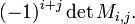$(-1)^{i+j} \det M_{i,j} . \,$

The adjugate matrix adj M (in older literature called adjoint matrix) is the n×n matrix whose (i,j) entry is the (j,i) cofactor (note the transposition of the indices). Letting In be the n×n identity (unit) matrix, we have$M \cdot \mathop{\mbox{adj}} M = \mathop{\mbox{adj}} M \cdot M = (\det M)\; I_n ,\,$

which encodes the rule for expansion of the determinant of M by any the cofactors of any row or column. This expression shows that if det(M) is non-zero, then M is invertible and its inverse is the following,$M^{-1} = (\det M)^{-1} \mathop{\mbox{adj}} M . \,$

## Example

Consider the following example matrix,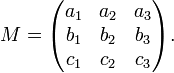$M = \begin{pmatrix} a_1 & a_2 & a_3 \\ b_1 & b_2 & b_3 \\ c_1 & c_2 & c_3 \\ \end{pmatrix}.$

Its minors are the determinants (vertical bars indicate a determinant):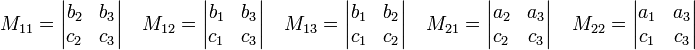$M_{11} = \begin{vmatrix} b_2 & b_3 \\ c_2 & c_3 \\ \end{vmatrix}\quad M_{12} = \begin{vmatrix} b_1 & b_3 \\ c_1 & c_3 \\ \end{vmatrix} \quad M_{13} = \begin{vmatrix} b_1 & b_2 \\ c_1 & c_2 \\ \end{vmatrix} \quad M_{21} = \begin{vmatrix} a_2 & a_3 \\ c_2 & c_3 \\ \end{vmatrix} \quad M_{22} = \begin{vmatrix} a_1 & a_3 \\ c_1 & c_3 \\ \end{vmatrix} \quad$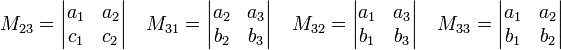$M_{23} = \begin{vmatrix} a_1 & a_2 \\ c_1 & c_2 \\ \end{vmatrix}\quad M_{31} = \begin{vmatrix} a_2 & a_3 \\ b_2 & b_3 \\ \end{vmatrix} \quad M_{32} = \begin{vmatrix} a_1 & a_3 \\ b_1 & b_3 \\ \end{vmatrix} \quad M_{33} = \begin{vmatrix} a_1 & a_2 \\ b_1 & b_2 \\ \end{vmatrix} \quad$

The adjugate matrix of M is$\mathrm{adj}M = A = \begin{pmatrix} M_{11} & -M_{21} & M_{31} \\ -M_{12} & M_{22} & -M_{32} \\ M_{13} & -M_{23} & M_{33} \\ \end{pmatrix},$

and the inverse matrix is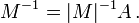$M^{-1} = |M|^{-1} A\, .$

Indeed,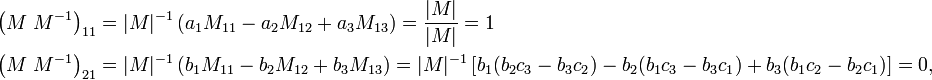\begin{align} \left( M\; M^{-1}\right)_{11} & = |M|^{-1}\left( a_1 M_{11}- a_2 M_{12} + a_3 M_{13}\right) = \frac{|M|}{|M|} = 1 \\ \left( M\; M^{-1}\right)_{21} & = |M|^{-1}\left( b_1 M_{11}- b_2 M_{12} + b_3 M_{13}\right) =|M|^{-1}\left[ b_1(b_2c_3-b_3c_2) - b_2(b_1c_3-b_3c_1) + b_3(b_1c_2-b_2c_1)\right] = 0 ,\\ \end{align}

and the other matrix elements of the product follow likewise.

## Note

1. The term "adjoint" for the adjugate matrix is disappearing because it is felt that it is easily confused with Hermitian adjoint, the transpose and complex conjugate of a matrix.